# The area of circle problems

#### Number of problems found: 183

• Circle simpleCircumference of a circle is 6.28. What is the area of the circle?
• Circle area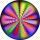Calculate the circle area with a radius of 1.2 m.
• Circle and rectangleA rectangle with sides of 11.7 cm and 175 mm is described by circle. What is its length? Calculate the content area of the circle described by this circle.
• Two annulusesThe area of the annular circle formed by two circles with a common center is 100 cm2. The radius of the outer circle is equal to twice the radius of the inner circle. Determine the outside circle radius in centimeters.
• CirclesArea of circle inscribed in a square is 14. What is the area of a circle circumscribed around a square?
• Three shapes1/5 of a circle is shaded. The ratio of area if square to the sum of area of rectangle and that of the circle is 1:2. 60% of the square is shaded and 1/3 of the rectangle is shaded. What is the ratio of the area of circle to that of the rectangle?
• Circle - simpleCalculate the area of a circle in dm2, if its circumference is 31.4 cm.
• Circular flowerbed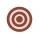Circular flowerbed with diameter 8 m we split by concentric circle to circle and annulus with the same area. Find the radius of the circle.
• Concentric circlesThere is given a circle K with a radius r = 8 cm. How large must a radius have a smaller concentric circle that divides the circle K into two parts with the same area?
• Squaring the Circle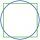Calculating side of the square with the same area as the circle of radius 18.
• CircleHow big is area of circle if its circumference is 80.6 cm?
• Circular ringSquare with area 16 centimeters square are inscribed circle k1 and described circle k2. Calculate the area of circular ring, which circles k1, k2 form.
• Diameter to areaFind the area of a circle whose diameter is 26cm.
• Equilateral triangle vs circle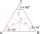Find the area of an equilateral triangle inscribed in a circle of radius r = 9 cm. What percentage of the circle area does it occupy?
• Circle in rhombus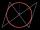In the rhombus is an inscribed circle. Contact points of touch divide the sides to parts of length 19 cm and 6 cm. Calculate the circle area.
• Diameters of circlesHow many percent of the area of a larger circle is a smaller circle if the smaller circle has a diameter 120 mm and a larger one has a diameter 300 mm?
• 22/7 circleCalculate approximately area of a circle with radius 20 cm. When calculating π use 22/7.
• Area to perimeterCalculate circle circumference if its area is 254.34cm2
• Circle arcCircle segment has a circumference of 135.26 dm and 2096.58 dm2 area. Calculate the radius of the circle and size of the central angle.
• Perimeter to areaCalculate the area of a circle with the perimeter 15 meters.

Do you have an interesting mathematical word problem that you can't solve it? Submit a math problem, and we can try to solve it.

We will send a solution to your e-mail address. Solved examples are also published here. Please enter the e-mail correctly and check whether you don't have a full mailbox.

Please do not submit problems from current active competitions such as Mathematical Olympiad, correspondence seminars etc...

Area - math word problems. Circle Problems.